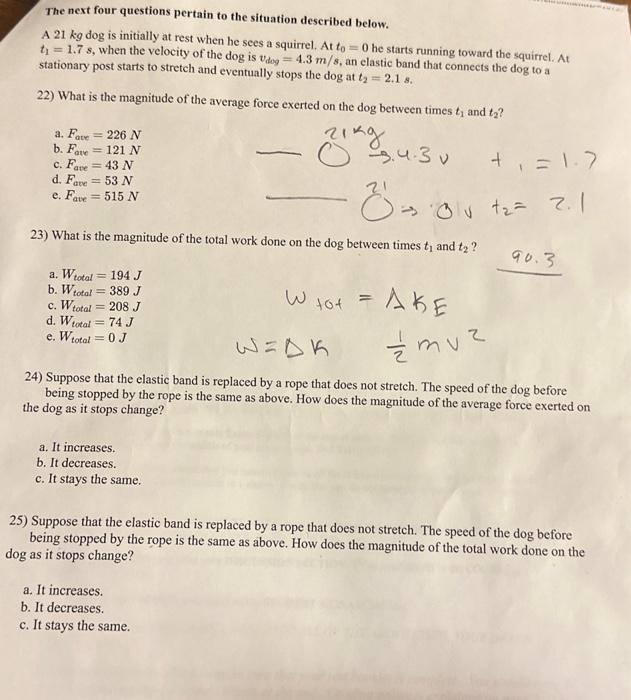Home / Expert Answers / Physics / can-you-help-me-with-22-25-the-next-four-questions-pertain-to-the-situation-described-below-a-21-pa912

# (Solved): Can you help me with 22-25? The next four questions pertain to the situation described below. A 21 ...

Can you help me with 22-25?The next four questions pertain to the situation described below. A dog is initially at rest when he sees a squirrel. At he starts running toward the squirrel. At , when the velocity of the is , an elastic band that connects the dog to a stationary post starts to stretch and eventually stops the dog at . 22) What is the magnitude of the average force exerted on the dog between times and ? a. b. c. d. e. 23) What is the magnitude of the total work done on the dog between times and ? a. b. c. d. e. 24) Suppose that the elastic band is replaced by a rope that does not stretch. The speed of the dog before being stopped by the rope is the same as above. How does the magnitude of the average force exerted on the dog as it stops change? a. It increases. b. It decreases. c. It stays the same. 25) Suppose that the elastic band is replaced by a rope that does not stretch. The speed of the dog before being stopped by the rope is the same as above. How does the magnitude of the total work done on the dog as it stops change? a. It increases. b. It decreases. c. It stays the same.

We have an Answer from Expert